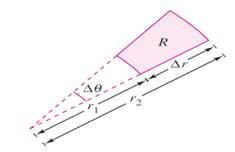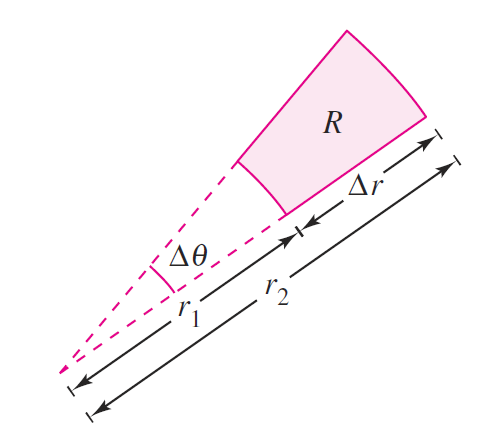Chapter 14.3, Problem 68E

Chapter
Section
Textbook Problem

AreaShow that the area of the polar sector R (see figure) is A = r Δ r Δ θ , where r = ( r 1 + r 2 ) 2 is the average radius of RTo determine

To Prove: The area of polar sector is A=rΔrΔθ, where r=(r1+r2)2 is the average radius of RExplanation

Given:

The polar sector:

r=(r1+r2)2

Formula Used:

The area of the sector is A=12r2θ

Calculation:

The area of the sector R is given by

A=Δθr222Δθr12

Still sussing out bartleby?

Check out a sample textbook solution.

See a sample solution

The Solution to Your Study Problems

Bartleby provides explanations to thousands of textbook problems written by our experts, many with advanced degrees!

Get Started

find the real roots of each equation by factoring. 132. 6x2 + x + 12 = 0

Applied Calculus for the Managerial, Life, and Social Sciences: A Brief Approach

CHECK POINT True or false: 6.

Mathematical Applications for the Management, Life, and Social Sciences

Evaluate the integral, if it exists. 0/4(1+tant)3sec2tdt

Single Variable Calculus: Early Transcendentals